Chapter Notes: Construction

# Construction Chapter Notes - Mathematics (Maths) Class 10

### Construction of a triangle similar to a given triangle as per the given scale factor when Scale Factor is more than 1

Q. Construct an isosceles triangle with base 5 cm and equal sides of 6 cm. Then, construct another triangle whose sides are of the corresponding sides of the (4/3)th of first triangle .

Steps of construction:
(i) Draw BC = 5 cm
(ii) With B and C as the centre and radius 6 cm, draw arcs on the same side of BC, intersecting at A.
(iii) Join AB and AC to get the required ΔABC.
(iv) Draw a ray , making an acute angle with BC on the side opposite to the vertex A.
(v) Mark 4 points B1, B2, B3, B4, along BX such that BB1 = B1B2 = B2B3 = B3B4
(vi) Join B3C. Draw a line through B4 parallel to B3C, making an angle equal to ∠BB3C intersecting the extended line segment BC at C´.
(vii) Through point C´, draw a line parallel to CA, intersecting extended BA at A´.
(viii) The resulting ΔA´BC´ is the required triangle.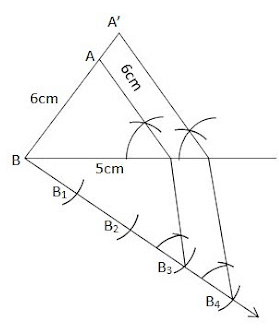### Division of a line segment in a given ratio

Q. Draw Line segment PQ=9cm and divide it in the ratio 2:5. Justify your construction.

Steps of construction:
(i) Draw Line Segment PQ=9cm
(ii) Draw a Ray PX, making an acute angle with PQ.
(iii) Mark 7 points A1 , A2 , A3 …A7 along PX such that PA1 = A3A2 = A2A3 = A3A4 = A4A5 = A5A6 = A6A7
(iv) Join QA7
(v) Through the point A2 , draw a line parallel to A7Q by making an angle equal to ∠PA7Q at A2, intersecting PQ at point R. PR:RQ = 2:5

Justification:

We have A2R || A7Q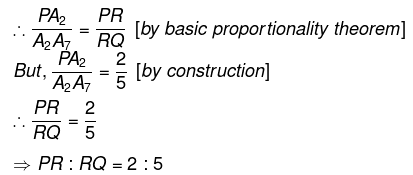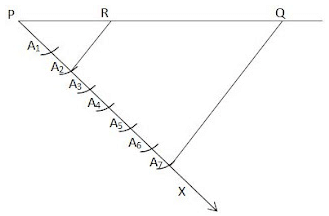### Construction of tangents to a circle

Q. Draw a circle of radius 3 cm. From a point 5 cm away from its centre, construct a pair

Steps of construction:
(i) Draw a circle with centre O and radius 3 cm. Take a point P such that OP = 5 cm, and then join OP.
(ii) Draw the perpendicular bisector of OP. Let M be the mid point of OP.
(iii) With M as the centre and OM as the radius, draw a circle. Let it intersect the previously drawn circle at A and B.
(iv) Joint PA and PB. Therefore, PA and PB are the required tangents. It can be observed that PA=PB=4cm.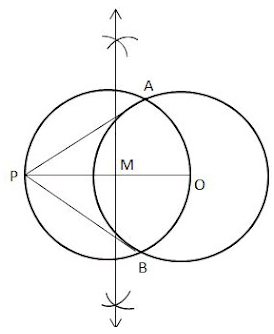### Construction of a triangle similar to a given triangle as per the given scale factor when Scale Factor is less than 1

Q. Draw a ΔABC with sides BC = 8 cm, AC = 7 cm, and ÐB = 70°. Then, construct a similar triangle whose sides are (3/5)th of the corresponding sides of the ΔABC.

Steps of construction:
(i) Draw BC = 8 cm
(ii) At B, draw ∠XBC = 70°
(iii) With C as centre and radius 7 cm, draw an arc intersecting BX at A.
(iv) Join AB, and DABC is thus obtained.
(v) Draw a ray , making an acute angle with BC.
(vi) Mark 5 points, B1, B2, B3, B4, B5, along BY such that
(vii) BB1 = B1B2 = B2B3 = B3B4 = B4B5
(viii) Join CB5
(ix) Through the point B3, draw a line parallel to B5C by making an angle equal to ∠BB5C, intersecting BC at C´.
(x) Through the point C´, draw a line parallel to AC, intersecting BA at A´. Thus, ΔA´BC´ is Required Triangle.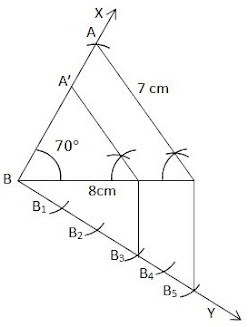Justification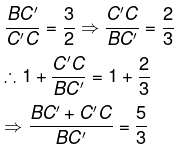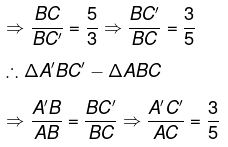Using BPT

The document Construction Chapter Notes | Mathematics (Maths) Class 10 is a part of the Class 10 Course Mathematics (Maths) Class 10.
All you need of Class 10 at this link: Class 10

## Mathematics (Maths) Class 10

115 videos|478 docs|129 tests

## FAQs on Construction Chapter Notes - Mathematics (Maths) Class 10

 1. What are the different types of construction materials commonly used in construction projects?Ans. The different types of construction materials commonly used in construction projects include concrete, steel, wood, brick, and glass. These materials have different properties and are used for various purposes in the construction industry.
 2. What is the importance of project planning in construction?Ans. Project planning is crucial in construction as it helps in organizing and managing resources efficiently, setting realistic timelines, and ensuring the smooth execution of a construction project. It allows for effective coordination between different stakeholders and helps in identifying potential risks and challenges in advance.
 3. What are the various stages involved in the construction process?Ans. The construction process typically involves several stages, including site preparation, foundation construction, structural framing, installation of utilities, interior and exterior finishing, and final inspections. Each stage requires careful planning and execution to ensure the successful completion of the project.
 4. What are some common safety measures that should be taken at construction sites?Ans. Safety measures at construction sites include providing personal protective equipment (PPE) to workers, implementing proper signage and barricades, conducting regular safety training programs, maintaining a clean and organized worksite, and adhering to all applicable safety regulations. It is important to prioritize the safety of workers and minimize the risk of accidents or injuries on construction sites.
 5. How does sustainable construction contribute to environmental conservation?Ans. Sustainable construction promotes the use of eco-friendly materials, energy-efficient designs, and green building practices, which help in minimizing the environmental impact of construction projects. It focuses on reducing waste, conserving natural resources, and creating healthier and more sustainable built environments. By adopting sustainable construction practices, we can contribute to environmental conservation and mitigate climate change.

## Mathematics (Maths) Class 10

115 videos|478 docs|129 testsExplore Courses for Class 10 examSignup to see your scores go up within 7 days! Learn & Practice with 1000+ FREE Notes, Videos & Tests.
10M+ students study on EduRev
Track your progress, build streaks, highlight & save important lessons and more!
Related Searches

,

,

,

,

,

,

,

,

,

,

,

,

,

,

,

,

,

,

,

,

,

;